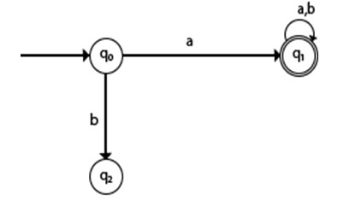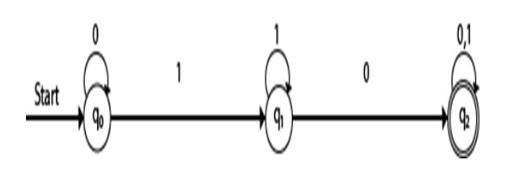# Explain Deterministic Finite Automata in TOC.

DFA refers to deterministic finite automata. Deterministic refers to the uniqueness of the computation. The finite automata are deterministic FA, if the machine reads an input string one symbol at a time.

In DFA, there is only one path input from the current state to the next state. It does not accept the null move, i.e. it cannot change state without any input. It can contain multiple final states. It is used in Lexical Analysis in compilers.

## Formal definition of different automata (DFA)

A Deterministic Finite automata (DFA) is a collection of defined as a 5-tuples and is as follows −

M=(Q, Σ, δ,q0,F)

Where,

• Q: Finite set called states.
• Σ: Finite set called alphabets.
• δ: Q × Σ → Q is the transition function.
• q0 ∈ Q is the start or initial state.
• F: Final or accept state.

## Graphical Representation of DFA

A DFA can be represented by digraphs called state diagrams.

The following factors are considered in DFA −

• The state is represented by vertices.
• The arc labelled with an input character shows the transitions.
• The initial state is represented with an arrow.
• The final state is represented by a double circle.

## Trap state in DFA

If a transition goes to a state from which it can never escape. Such a state is called a trap state. It is called the dead state.In the above example, q2 is a trap or dead state because it can’t reach the final state.

## Application of DFA (Deterministic Finite Automata)

The different applications of deterministic finite automata are as follows −

• Protocol analysis text parsing.
• Video game character behavior.
• Security analysis.
• CPU control units.
• Natural language processing Speech recognition, etc.

## Example

Construct transition table of DFA for a given diagram.

Q= {q0,q1,q2}

Σ ={0,1}

q0= {q0}

F= {q2}

## Transition diagram

The transition diagram is as follows −## Transition Table

The transition table is as follows −

Present stateNext state for input 0Next state for input 1
->q0q0q1
q1q2q1
*q2q2q2

## Explanation

• Step 1 − In the above table q0 is the initial state, on input ‘0’ the q0 state goes to itself and on input ‘1’ it goes to state q1.
• Step 2 − q1 is the intermediate state, on input ‘0’ q1 goes to q2 state and on input ‘1’, q1 goes to itself.
• Step 3 − q2 is the final state, q2 on ‘0’ goes to q2 itself and input ‘1’ goes to q2 itself.